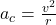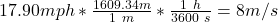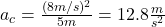## A 3.0 X 10^3 grams rock swings in a circle with a diameter of 1000 cm. Given the constant speed around the circle is 17.90 mph (a) what is t

Question

A 3.0 X 10^3 grams rock swings in a circle with a diameter of 1000 cm. Given the constant speed around the circle is 17.90 mph (a) what is the centripetal acceleration. *

in progress 0
5 months 2021-09-05T09:21:50+00:00 1 Answers 2 views 0

a = 12.8 m/s^2

Explanation:

To find the centripetal acceleration you use the following formula:(1)

v: tangential speed of the rock = 17.90mph

r: radius of the orbit = 1000cm/2 = 500cm = 0.5m

You first change the units of the tangential velocity in order to replace the values of v and r in the equation (1):Then, you use the equation (1):hence, the centripetal acceleration is 12.8 m/s^2